• ## 逻辑回归算法原理

万次阅读 2018-05-13 21:26:25
逻辑回归也被称为对数几率回归，算法名虽然叫做逻辑回归，但是该算法是分类算法，个人认为这是因为逻辑回归用了和回归类似的方法来解决了分类问题。 逻辑回归模型是一种分类模型，用条件概率分布的形式表示 P(Y|X)P...
http://ihoge.cn/2018/LR.html

逻辑回归模型

逻辑回归也被称为对数几率回归，算法名虽然叫做逻辑回归，但是该算法是分类算法，个人认为这是因为逻辑回归用了和回归类似的方法来解决了分类问题。

逻辑回归模型是一种分类模型，用条件概率分布的形式表示 P(Y|X)$P\left(Y|X\right)$$P(Y|X)$，这里随机变量 X 取值为 n 维实数向量，例如x=(x(1),x(2),...,x(n))$x=\left({x}^{\left(1\right)},{x}^{\left(2\right)},...,{x}^{\left(n\right)}\right)$$x=(x^{(1)},x^{(2)},...,x^{(n)})$，Y 取值为 0 或 1。即：
P(Y=1|x)=exp(w⋅x+b)1+exp(w⋅x+b)$P\left(Y=1|x\right)=\frac{\mathrm{exp}\left(w\cdot x+b\right)}{1+\mathrm{exp}\left(w\cdot x+b\right)}$P\left( Y=1 \right|x)=\frac{\exp \left( w\cdot x+b \right)}{1+\exp \left( w\cdot x+b \right)}

P(Y=0|0)=11+exp(w⋅x+b)$P\left(Y=0|0\right)=\frac{1}{1+\mathrm{exp}\left(w\cdot x+b\right)}$P\left( Y=0 \right|0)=\frac{1}{1+\exp \left( w\cdot x+b \right)}

或：

ϕ(x)=11+e−wTx−b$\varphi \left(x\right)=\frac{1}{1+{e}^{-{w}^{T}x-b}}$\phi \left( x \right)=\frac{1}{1+e^{-w ^{T}x-b}}

假设有一个二分类问题，输出为y∈{0,1}$y\in \left\{0,1\right\}$$y\in \left\{ 0,1 \right\}$，二线性回归模型z=wTx+b$z={w}^{T}x+b$$z=w^Tx+b$是个实数值，我们希望有一个理想的阶跃函数来帮我什么实现z值到0/1值的转化，于是找到了Sigmoid函数来代替：
g(z)=11+e−z$g\left(z\right)=\frac{1}{1+{e}^{-z}}$g\left( z \right)=\frac{1}{1+e^{-z}}有了 Sigmoid 函数之后，由于其值取值范围在[0,1]。就可以将其视为类 1 的后验概率估计 p(y=1|X)$p\left(y=1|X\right)$$p(y=1|X)$。说白了，就是如果有了一个测试点 x，那么就可以用Sigmoid函数算出来的结果当作该点 x 属于类别 1 的概率大小。

于是，非常自然地，我们把 Sigmoid 函数计算得到的值大于等于0.5的归为类别1，小于0.5的归为类别0：

逻辑函数的损失函数

接下来要做的就是根据给定的训练集，把参数 w 给求出来了。要找参数 w，首先就得把代价函数（Cost Function）给定义出来，也就是目标函数。

我们第一个想到的自然是模仿线性回归的做法，利用误差平方和来当代价函数:
J(θ)=12m∑i=0m(ϕ(xi)−yi)2$J\left(\theta \right)\phantom{\rule{thickmathspace}{0ex}}=\phantom{\rule{thickmathspace}{0ex}}\frac{1}{2m}\sum _{i=0}^{m}{\left(\varphi \left({x}^{i}\right)-{y}^{i}\right)}^{2}$J\left( \theta  \right)\; =\; \frac{1}{2m}\sum_{i=0}^{m}{\left( \phi \left( x^{i} \right)-y^{i} \right)^{2}}
这时将预测函数g(z(i))=11+e−x(i)$g\left({z}^{\left(i\right)}\right)=\frac{1}{1+{e}^{-{x}^{\left(i\right)}}}$$g\left( z^{\left( i \right)} \right)=\frac{1}{1+e^{-x^{\left( i \right)}}}$代入损失函数的话，会发现这是一个非凸函数，这意味着代价函数有着许多的局部最小值，这不利于我们求解：那么我们不妨来换一个思路解决这个问题。前面，我们提到了 ϕ(z) 可以视为类1的后验估计，所以我们有：其中 p(y=1|x;w)$p\left(y=1|x;w\right)$$p(y=1|x;w)$ 表示给定 w，那么 x 点 y=1 的概率大小。于是上面两式可以写成一般形式：注：以上的过程说明，最大似然估计与误差平方和等价！这就是为什么逻辑回归的损失函数可以用最大似然函数进行估计的原因。

接下来我们就要用极大似然估计来根据给定的训练集估计出参数 w：为了简化运算，我们对上面这个等式的两边都取一个对数：我们现在要求的是使得 l(w) 最大的 w。没错，我们的代价函数出现了，我们在 l(w) 前面加个负号不就变成就最小了吗？不就变成我们代价函数了吗？为了更好地理解这个代价函数，我们不妨拿一个例子的来看看：也就是说：下面是函数图：从图中不难看出，如果样本的值是1的话，估计值 ϕ(z) 越接近1付出的代价就越小，反之越大；同理，如果样本的值是0的话，估计值 ϕ(z) 越接近0付出的代价就越小，反之越大。

逻辑回归的模型求解

在开始梯度下降之前，要这里插一句，Sigmoid function 有一个很好的性质就是 ：
ϕ′(z)=ϕ(z)(1−ϕ(z))${\varphi }^{\prime }\left(z\right)=\varphi \left(z\right)\left(1-\varphi \left(z\right)\right)$\phi '\left( z \right)=\phi \left( z \right)\left( 1-\phi \left( z \right) \right)
这个后续会用到。

还有，我们要明确一点，梯度的负方向就是代价函数下降最快的方向：这里来解释下。借助泰勒公式展开，有：其中，f′(x) 和 δ 为向量，那么这两者的内积就等于：当 θ=π 时，也就是 δ 在 f′(x) 的负方向上时，取得最小值，也就是下降的最快的方向了。
于是有：即：其中，wj${w}_{j}$$w_j$ 表示第 j 个特征的权重；η 为学习率，用来控制步长。 重点来了:所以，在使用梯度下降法更新权重时，只要根据下式即可：此式与线性回归时更新权重用的式子极为相似，也许这也是逻辑回归要在后面加上回归两个字的原因吧。当然，在样本量极大的时候，每次更新权重会非常耗费时间，这时可以采用随机梯度下降法，这时每次迭代时需要将样本重新打乱，然后用下式不断更新权重：也就是去掉了求和，而是针对每个样本点都进行更新。
展开全文LR 机器学习
• 一、逻辑回归概述逻辑回归的名字里虽然有回归二字，却不是回归算法，只是保留了回归算法的原理，逻辑回归主要是用来处理分类问题，包括二元...二、手推逻辑回归算法首先引入sigmoid函数： ，对 导数手推过程如下（...
一、逻辑回归概述逻辑回归的名字里虽然有回归二字，却不是回归算法，只是保留了回归算法的原理，逻辑回归主要是用来处理分类问题，包括二元回归以及多元回归。在线性回归中，我们输出的值都是连续型的，那我们能不能通过一种转化来处理分类问题呢，比如在某一种区间输出为一种类别，而在另一种区间输出为另一种类别，逻辑回归的原理就是这样来的。二、手推逻辑回归算法首先引入sigmoid函数：，对导数手推过程如下（字不好看，见谅呀）：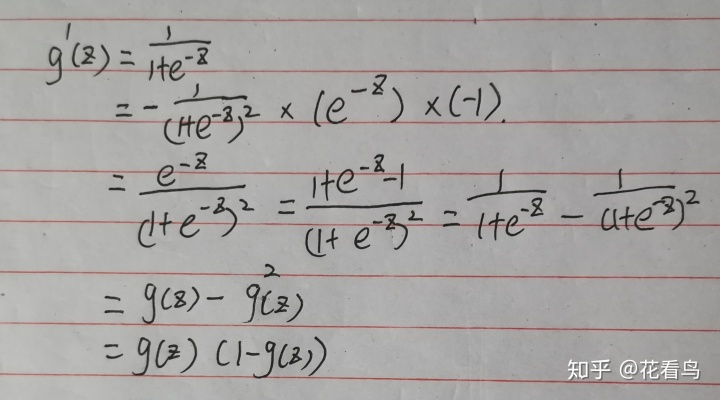当时，可以得出二元回归模型的一般形式：，当时，此时，y取1，当时，此时，y取0，当时，无法判断分类，推导的主要原理是对函数进行对数转化再取反，过程如下：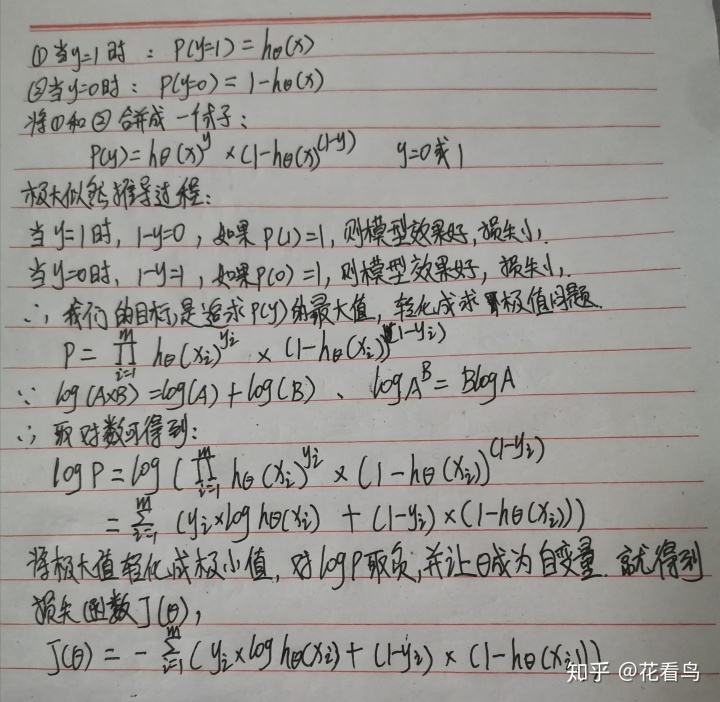这里简单说明一下概率和似然的区别：概率是指因变量和自变量的关系似然是指因变量和参数的关系三、逻辑回归的优化过程对损失函数的自变量求偏导，可以得到：由梯度下降法求解出：四、逻辑回归正则化逻辑回归的正则化有L1正则化和L2正则化。L1正则化：损失函数+，其中为的范式L2正则化：损失函数+，其中为的范式越大正则化强度越大，其中一般不需要正则化。五、逻辑回归优点对线性关系比较强的拟合效果好抗噪声能力强计算快
展开全文• 逻辑回归原理逻辑回归算法是数据挖掘中经常被用到的分类方法，尤其对于两类分类问题。逻辑回归算法的一个优势就是它是基于概率的分类算法并且很容易被扩展到多类问题。更重要的是大多数无约束最优化技术都可以应用到...
逻辑回归原理逻辑回归算法是数据挖掘中经常被用到的分类方法，尤其对于两类分类问题。逻辑回归算法的一个优势就是它是基于概率的分类算法并且很容易被扩展到多类问题。更重要的是大多数无约束最优化技术都可以应用到逻辑回归的求解过程中。在逻辑回归算法中对数似然比由线函数来拟合。对于两类的情况，可用式(2.1)表示：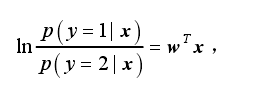（3-1）其中，x  代表实例，w 代表未知的参数，w 的选择应该使得实例属于各个类的概率之和等于 1，即：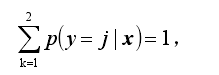(3-2)联合(2.1)(2.2)可以得到，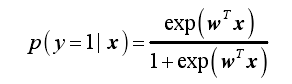(3-3)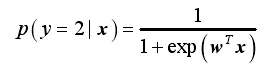(3-4)w 可以通过最大似然估计进行计算，其对数似然函数为：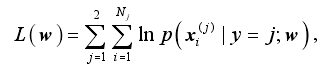(3-5)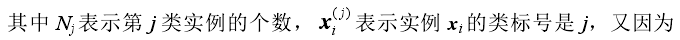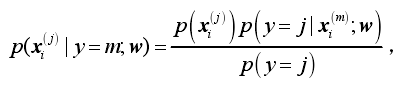(3-6)所以目标函数可写成：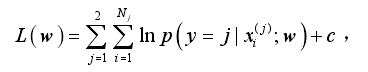(3-7)其中，c 表示一个常量。 从式(2.5)可以看出，使用最大似然估计方法求解逻辑判别中参数 w 的目标是使学习到的模型正确分类各个实例的概率对数和最大化，而不考虑该实例是多数类实例还是少数类实例。《来源科技文献，经本人分析整理，以技术会友，广交天下朋友》
展开全文• 逻辑回归假设数据服从伯努利分布,通过极大化似然函数的方法，运用梯度下降来求解参数，来达到将数据二分类的目的逻辑回归算法是从统计学借鉴过来的，虽然字面意思里面有回归，但是更多用于分类。也是常用的分类机器...
逻辑回归假设数据服从伯努利分布,通过极大化似然函数的方法，运用梯度下降来求解参数，来达到将数据二分类的目的逻辑回归算法是从统计学借鉴过来的，虽然字面意思里面有回归，但是更多用于分类。也是常用的分类机器学习算法。假设模型为：癌症检测算法可看做是 Logistic 回归问题的一个简单例子 我们需要通过分类器预测分类结果：是（记为1）or不是（记为0）。如果我们考虑0就是“不发生”，1就是“发生”，那么我们可以将分类任务理解成估计事件发生的概率p， 通过事件发生概率的大小来达到分类目的。因此，我们需要使得预测结果即概率值限定在0～1之间，很明显，如果我们仍然采用线性回归p=fθ(x)=θTx作为分类器，其预测值可能会远远大于1或者远远小于0，不符合我们预期想法。所以，逻辑回归引入logistic函数，将分类器输出界定在［0，1］之间，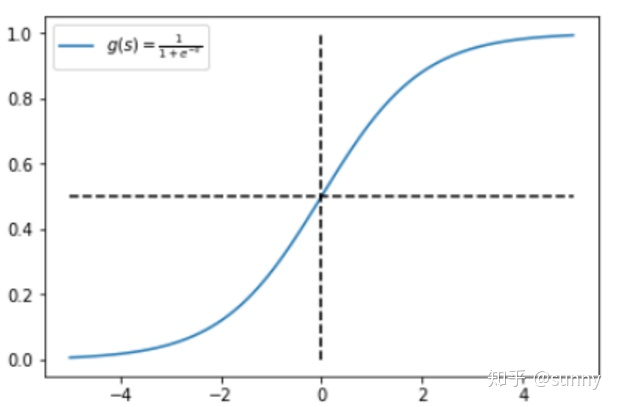hθ(x)的作用是，对于给定的输入变量，根据选择的参数计算输出变量=1 的可能性（estimated probablity）即也是我们的目标函数以上只是得出了样本点是正例的概率，到底预测它是正例还是负例，我们还需要一个decision boundary，例如由于sigmoid函数的形状我们容易得到当然，decision boundary属于假说函数的一部分，不一定就是0.5逻辑回归的损失函数(交叉熵函数)损失函数，通俗讲，就是衡量真实值和预测值之间差距的函数。所以，我们希望这个函数越小越好。在这里，最小损失是0。交叉熵函数的推导过程逻辑回归中最大损失函数到底是怎么来的，因为我看到很多地方只是说了一下用到最大似然的方法。Ng直接给了损失函数 既然是最大似然，我们的目标当然是要最大化似然概率了：其中θ是未知参数。假设有一组观测样本，那么现在的任务就变成给定一组数据和一个参数待定的模型下，如何估计模型参数的问题。而这里想要讲述的最大似然估计就是估计未知参数的一种方法，最大似然估计（Maximum Likelihood Method）是建立在各样本间相互独立且样本满足随机抽样（可代表总体分布）下的估计方法，它的核心思想是如果现有样本可以代表总体，那么最大似然估计就是找到一组参数使得出现现有样本的可能性最大，即从统计学角度需要使得所有观测样本的联合概率最大化，又因为样本间是相互独立的，所以所有观测样本的联合概率可以写成各样本出现概率的连乘积，如下式：为计算方便，取对数，得到我们希望 log P(y|x) 越大越好，反过来，只要 log P(y|x) 的负值 -log P(y|x) 越小就行了。那我们就可以引入损失函数，且令 Loss = -log P(y|x)即可。则得到损失函数为优化过程梯度下降更新算法又这就得到了逻辑回归的梯度下降更新式子参考如下：逻辑回归的常见面试点总结https://www.cnblogs.com/ModifyRong/p/7739955.html机器学习入门 - 快速掌握逻辑回归模型http://blog.itpub.net/29829936/viewspace-2558236/从原理到应用：简述Logistics回归算法https://www.jiqizhixin.com/articles/2018-05-13-3逻辑回归与最大似然估计 http://www.hanlongfei.com/机器学习/2015/08/05/mle/
展开全文• 一、什么是逻辑回归在前面讲述的回归模型中，处理的因变量都是数值型区间变量，建立的模型描述是因变量的期望与自变量之间的线性关系。比如常见的线性回归模型：而在采用回归模型分析实际问题中，所研究的变量往往不...
• 逻辑回归属于经典的二分类算法，但是为什么叫回归呢？是因为逻辑回归可以通过sigmoid函数，将线性回归的结果（数值）映射为概率，有了概率，就可以根据概率大小判断属于哪一类。Sigmoid函数公式及其几何表示可以将...
• 本课程讲解现在工业界和学术界流行的机器学习算法的相关知识，以及如何用python去实现这些算法。...其中supervised learning包括classification(分类)和regression(回归)的若干流行的模型的讲解。 Unsupervised l...
• 逻辑回归算法原理及用于解决多分类问题 逻辑回归算法又叫做对数几率回归，作为一种分类算法，在统计学中属于广义的线性模型。实际上逻辑回归是通过对数几率函数将线性函数的结果进行mapping，这样一来目标函数的取值...机器学习 人工智能
• 本文主要讨论二阶优化算法逻辑回归只是一个用来帮助实现的手段而已，不会过多讨论。在之前的文章中有介绍过神经网络的基本单元——神经元永远在你身后：Numpy实现神经网络框架(1)​zhuanlan.zhihu.com不考虑激活...
• 逻辑回归完成分类任务，是经典的二分类算法（也可以解决多分类问题），机器学习算法一般选择先逻辑回归（优先选择）再用复杂的，逻辑回归的决策边界可以是非线性的（高阶）。可以通过参数对结果进行评估。 2，...
• 1.逻辑回归原理 逻辑回归算法（Logistic Regression）是解决分类问题，是将样本的特征和样本发生的概率联系起来，而概率是一个数，所以 2.逻辑回归算法推导 3.基于Python的代码实现 ...
• 逻辑回归算法公式计算5.算法python实现1.什么是逻辑回归逻辑回归的过程可以概括为：面对一个回归或者分类问题，建立代价函数，然后通过优化方 法迭代求解出最优的模型参数，然后测试验证我们这个求解的模型的好坏。 ...
• 机器学习算法选择：先逻辑回归再用复杂的，能简单还是用简单的 逻辑回归的决策边界：可以是非线性的 Sigmoid函数 公式：g(z)=11+e−zg(z)=\frac{1}{1+e^{-z}}g(z)=1+e−z1​ 自变量取值为任意实数，值域为[0，1] ...
• 【机器学习】算法原理详细推导与实现(二):逻辑回归在上一篇算法中，线性回归实际上是 连续型 的结果，即 ，而逻辑回归的 是离散型，只能取两个值 ，这可以用来处理一些分类的问题。logistic函数我们可能会遇到一些...
• 逻辑回归算法公式计算5.算法python实现1.什么是逻辑回归逻辑回归的过程可以概括为：面对一个回归或者分类问题，建立代价函数，然后通过优化方 法迭代求解出最优的模型参数，然后测试验证我们这个求解的模型的好坏。 ...
• 逻辑回归算法原理推导 Logistic Regression和Linear Regression的原理是相似，但是做的事情不同，Linear Regression解决的是一个具体确定值的问题，Logistic Regression解决的是分类的问题，而且是最经典的二分法...LR
• 1.逻辑回归原理 1.决策边界 梯度下降要做的就是在使损失函数尽量小的情况下求的一组Θ 2.损失函数 为何此处损失函数要用对数损失？ https://www.jianshu.com/p/b6bb6c035d8c 2.线性逻辑回归python实现 数据集...
• 算法原理：  逻辑回归是一个流行的二分类问题预测方法。它是Generalized Linear models 的一个特殊应用以预测结果概率。它是一个线性模型如下列方程所示，其中损失函数为逻辑损失：    对于二分类问题，算法...MLlib 数据挖掘 机器学习 Spark
• 逻辑回归算法公式计算5.算法python实现1.什么是逻辑回归逻辑回归的过程可以概括为：面对一个回归或者分类问题，建立代价函数，然后通过优化方 法迭代求解出最优的模型参数，然后测试验证我们这个求解的模型的好坏。 ...
• 逻辑回归(Logistic Regression)是一个非常经典的算法.逻辑回归用来处理不同的分类问题，目的是预测当前被观察对象属于哪个组，它会提供一个离散的二进制输出结果.因其简单、可并行化、可解释强深受工业界喜爱。逻辑...逻辑回归阈值
• 逻辑回归 简介 在一元回归和多元回归模型中，处理的因变量都是数值型区间变量，建立的模型描述的是因变量的期望与自变量之间的线性关系。然而，在实际的问题分析时，所研究的变量往往不全是区间变量而是顺序变量...
• 优点：原理简单，容易实现，应用广泛机器学习算法的选择：先逻辑回归，再用复杂的。决策边界：可以是非线性的。 Sigmoid函数 自变量取值为任意实数，值域[0,1] 将任意的输入映射到[0,1]区间，再在线性回归中...人工智能
• 逻辑回归算法用于估计预测目标的可能性，它属于软分类算法，即最终得到的是一个具体的概率，而不仅仅是“是”或“不是”这样的二分类结果；逻辑回归能提供一个样本属于正类的可能性是多少，比如假设对于样本x，回归...人工智能 机器学习 伪代码
• 线性回归算法是机器学习中最基本的一个算法，但是该算法的思想、原理相当重要。本文将详细从原理上（用似然函数）讲解线性回归算法 从一个例子引入：想象一下，假如我要去银行贷款，银行会问我两个问题，一是工资...机器学习 线性回归 最小二乘法...